Courses

# Mathematics: CBSE Sample Question Paper (2020-21) (Basic) - 4 Notes | EduRev

## Class 10 : Mathematics: CBSE Sample Question Paper (2020-21) (Basic) - 4 Notes | EduRev

The document Mathematics: CBSE Sample Question Paper (2020-21) (Basic) - 4 Notes | EduRev is a part of the Class 10 Course CBSE Sample Papers For Class 10.
All you need of Class 10 at this link: Class 10

Class X
Mathematics – Basic
Sample Question Paper 2020-21
Max. Marks : 80
Duration : 3 hrs.

General Instructions :

1. This question paper contains two parts A and B.
2. Both Part A and Part B have internal choices.
Part – A
1. It consists of two sections - I and II.
2. Section I has 16 question. Internal choice is provided in 5 questions.
3. Section II has four case study-based questions. Each case study has 5 case-based sub-parts. An examinee is to attempt any 4 out of 5 sub-parts.
Part – B
1. Question No 21 to 26 are Very short answer Type questions of 2 marks each.
2. Question No 27 to 33 are Short Answer Type questions of 3 marks each.
3. Question No 34 to 36 are Long Answer Type questions of 5 marks each.
4. Internal choice is provided in 2 questions of 2 marks, 2 questions of 3 marks and 1 question of 5 marks.

Part - A

Section - I

Q.1. If two positive integers a and b are written as a = x3y3 and b = x4y3; x, y are prime numbers, then find the value of HCF (a, b).  (1 Mark)
Ans. Since a = x3y3 = (x).(x).(x).(y).(y).(y) and
b = x4y3 = (x).(x).(x).(x).(y).(y).(y)
Therefore,
HCF of a and b = x3y3.

Q.2. Find the sum of the zeroes of the polynomial 5x2 – 30x + 1.  (1 Mark)
OR
Given that one of the zeroes of the cubic polynomial ax3 + bx2 + cx + d is zero, then find the product of the other two zeroes.  (1 Mark)

Ans. Sum of zeros = − (Coefficient of x) / (Coefficient of x2) = -(-30) / 5 = 6
OR
Let f(x) = ax3 + bx2 + cx + d If a, b, g are the zeroes of f(x), then
αβ + βγ+ γα = c / a
One root is zero (given) so, a = 0. βγ = c / a.

Q.3. In an A.P., if d = – 5, n =125, a = 4, then find the value of an.  (1 Mark)
Ans. In the given A.P., d = – 5, n =125, a = 4
an = a+(n – 1) d
= 4 + (125 – 1)(– 5)
= 4 – 620
∴ a125 = – 616

Q.4. Find the distance between the points A (4, 3) and B (– 4, – 3).  (1 Mark)
Ans. By distance formula, distance between (x1, y1) and (x2, y2) is given by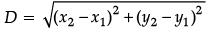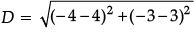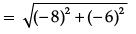√100 = 10

Q.5. Find the value of (sin 30° + cos 30°) – (sin 60° + cos 60°) is.  (1 Mark)
Ans. (sin 30° + cos 30°) – (sin 60° + cos 60°)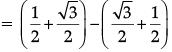= 0

Q.6. Find the area of the square that can be inscribed in a circle of radius 8 cm.  (1 Mark)
Ans.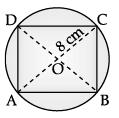Given, radius of circle, r = OC = 8 cm
Diameter of the circle = AC = 2 × OC = 2 × 8 = 16 cm which is equal to the diagonal of a square.
Let side of square be a cm
Using Pythagoras theorem in ΔABC,
AB2 + BC2 = AC2
a2 + a2 = 162
2a2 = 256
a2 = 128 cm2

Q.7. Write the first four terms of an A.P., whose first term is –2 and the common difference is –2.  (1 Mark)
Ans. In the given A.P., a = – 2, d = – 2,
tn = a + (n - 1)d
t1 = (-2) + (1 - 1) (-2) = -2
t2 = (-2) + (2 - 1) (-2) = -4
t3 = (-2) + (3 - 1) (-2) = -6
t4 = (-2) + (4 - 1) (-2) = -8

Q.8. If 4 tan θ = 3, then find the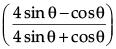.  (1 Mark)
OR
If tan (3x + 30°) = 1, then find the value of x.  (1 Mark)

Ans. Given, 4 tan θ = 3
⇒ tan θ = 3 / 4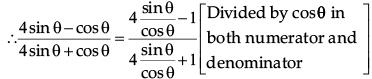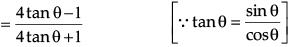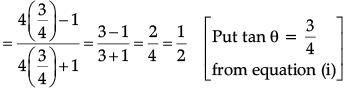OR
tan (3x + 30°) = 1 = tan 45°
or, 3x + 30° = 45° or, x = 5°

Q.9. Find the HCF of 75 and 125.  (1 Mark)
Ans.
75 = 5× 5 × 3 and 125 = 5 × 5 × 5 HCF(75, 125) = 25.

Q.10. If the quadratic polynomial is 4x2 + 8x, then find product of zeroes.  (1 Mark)
Ans. For quadratic polynomial ax2 + bx + c,
Product of zeroes, α × β = (c / a)
Therefore, for 4x2 + 8x +0
Product of zeroes = 0 / 4 = 0.

Q.11. If two angles of one triangle are respectively equal to two angles of another triangle, then the two triangles are similar by ……… test of similarity.  (1 Mark)
Ans. AA (angle-angle)test of similarity.

Q.12. A ……. to a circle is a line that intersects the circle at one point only.  (1 Mark)
Ans. A ‘TANGENT ’ to a circle is a line that intersects the circle at one point only.

Q.13. Find the mean of observations 2, 3, 5, 6, 7.  (1 Mark)
OR
The mean of n quantities x1, x2, x3, ... xn is ............. .  (1 Mark)

Ans.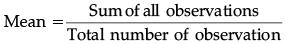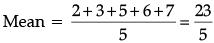or, Mean = 4.6
OR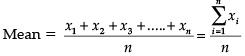Q.14. If the pair of linear equations given by 4x – 2y = 12 and – kx + 4y = –24 is dependent and consistent, then find the value of k.  (1 Mark)
OR
If the distance between the points (p, –1) and (4, –5) is √41 , then find the value of p.  (1 Mark)

Ans. Condition for dependent and consistent :
a1 / a2 = b1 / b2 = c1 / c2
∴ for 4x – 2y = 12 and – kx + 4y = – 24
4 / -k = -2 / 4 = -12 / 24
∴ 2k = 16 or k = 8
OR
By distance formula, distance between (x1, y1) and (x2, y2) is given by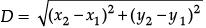(x1, y1) = (p, – 1) and (x2, y2) = (4, – 5)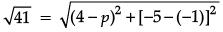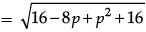∴ p2 – 8p + 32 = 41
p2 – 8p – 9 = 0
(p – 9)(p + 1) = 0
∴ p = 9 or p = – 1

Q.15. In the figure, PQ is parallel to MN. If KP : PM = 4 : 13 and KN = 20.4 cm, then find KQ.   (1 Mark)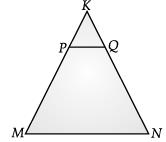Ans. ∵ PQ || MN
So, KP / PM = KQ / QN  (By BPT)
or, KP / PM = KQ / KN - KQ
or, 4 / 13 = KQ / 20.4 - KQ
or, 4 ×  20.4 – 4 KQ = 13 KQ
or, 17 KQ = 4 × 20.4
∴ KQ =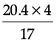= 4.8 cm.

Q.16. If the circumferences of two concentric circles forming a ring are 88 cm and 66 cm respectively. Find the width of the ring.   (1 Mark)
OR
What is the name of a line which intersects a circle at two distinct points?  (1 Mark)

Ans. ∵ Circumference of the outer circle, 2πr1 = 88 cm
∴ r1 = 88 x 7 / 22 x 2 = 14 cm.
∴ Circumference of the inner circle, 2πr2 = 66 cm
∴ r2 = 66 x 7 / 22 x 2 = 21 / 2 cm = 10.5 cm
∴ Width of the ring = r1 – r2
= 14 – 10.5 cm = 3.5 cm.
OR
A line intersecting the circle at two distinct points is called a secant.

Section - II

Q.17. The given figure, shows the arrangement of desks in a classroom. Ashima, Bharti and Camella are seated at A (3, 1), B (6, 4) and C (8, 6) respectively.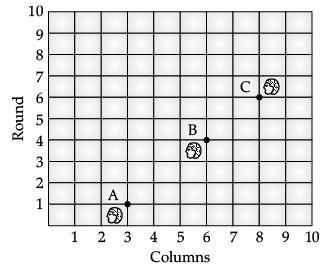(i) Find the distance between Ashima and Bharti.  (1 Mark)
(a) 2√3 units
(b) 3√2 units
(c) √2 units
(d) √3 units
Ans. (b)
Solution. Distance between Ashima and Bharti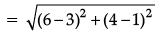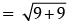√18 = 3√2 units

(ii) Find the distance between Bharti and Camella.  (1 Mark)
(a) 2√2 units
(b) √2 units
(c) √3 units
(d) 3√2 units
Ans. (a)
Solution. Distance between Bharti and Camella.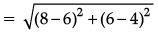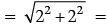√8 = 2√2 units

(iii) At how much distance Ashima is seated from Camella?  (1 Mark)
(a) 2√5 units
(b) 5√2 units
(c) 2 units
(d) 5 units
Ans. (b)
Solution. Distance between Ashima and Camella.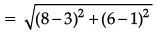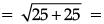√50 = 5√2 units

(iv) Write the formula for distance between : (x1, y1) and (x2, y2)  (1 Mark)
(a)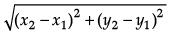(b)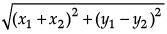(c)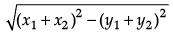(d) (x1 + x2)2 + (y1 - y2)
2
Ans. (a)
Solution. Distance formula for two points (x1, y1) and (x2, y2)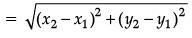(v) Write the formula for mid-points of two points :  (1 Mark)
(a)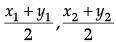(b)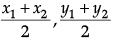(c)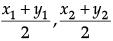(d)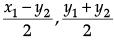Ans. (b)
Solution.
Mid points =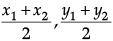.

Q.18. There is a small island in the middle of 100 m wide river and a tall tree stands on the island. A and B are points directly opposite to each other on two banks and in line with the tree.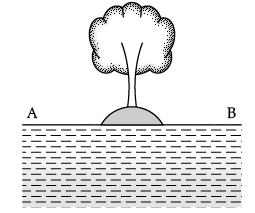(i) It the angles of elevation of the top of the tree from A and B are respectively 45° and 60°. Find the height of tree.  (1 Mark)
(a) 50 (3 − √3)
(b) 50 (3 − √3)
(c) 50 (3 + √3)
(d) 50 (√3 +3)
Ans. (a)
Solution. In ΔAPD,
tan 45° = PD / AD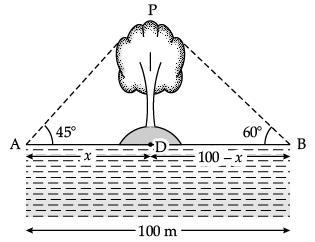1 = PD / x
PD = x ...(i)
In ΔBPD,
tan 60° = PD / BD
√3 = PD / 100 − x
√3 (100 – x) = PD ...(ii)
Equating (i) and (ii)
x = √3 (100 – x)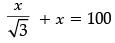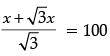x (√3 +1) = 100√3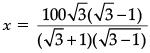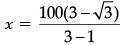= 50 (3 − √3) m
Height of tree, PD = x = 50 (3 − √3) m

(ii) Find the distance from A to tree.  (1 Mark)
(a) 50 ( 3 − √3) m
(b) 51 (3 − √2) m
(c) 50 (2 − √3) m
(d) 25 (2 − √3) m.
Ans. (a)
Solution. AD = x = 50 (3 − 3) m.

(iii) Find the distance from B to tree.  (1 Mark)
(a) 50 (3 − √1) m
(b) 25 (3 − √1) m
(c) 50 (3 + √1) m
(d) 25 (3 − √1) m
Ans. (a)
Solution. BD = 100 – x
= 100 – 50 (3 − 3)
= 50 (2 − 3 + 3)
= 50 (3 − 1) m.

(iv) The value of tan 45° is equal to :  (1 Mark)
(a) 0
(b) 1
(c) 2
(d) √3 / 2

Ans. (b)
Solution. tan 45° = 1.

(v) The value of tan 60° is equal to :  (1 Mark)
(a) 1 / √3
(b) √3
(c) 1 / √2
(d) √3 / 2

Ans. (b)
Solution. tan 60° = 3.

Q.19. Sheena has made a circular table cover with a hand knit embroidery on it. She made triangular designs on table cloth. In a circular table cover of radius 32 cm, a design is formed leaving an equilateral triangle ABC in the middle as shown in the given figure.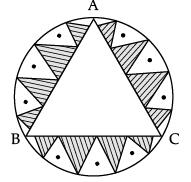(i) Find the circumference of circular table cover.  (1 Mark)
(a) 200.50 cm
(b) 201.2 cm
(c) 201.14 cm
(d) 220.15 cm
Ans. (c)
Solution. Circumference of table Cover = 2πr.
= 2 x 22 / 7 x 32 cm
= 201.14 cm.

(ii) Find the diameter of circle having radius 32 cm.  (1 Mark)
(a) 60 cm
(b) 65 cm
(c) 62 cm
(d) 64 cm
Ans. (d)
Solution. Diameter = 2 × radius
= 2 × 32 cm
= 64 cm.

(iii) Find the area of the circle (see the figure).  (1 Mark)
(a) 3281.25 cm2
(b) 3250.20 cm2
(c) 3261.5 cm2
(d) 3218.28 cm2
Ans. (d)
Solution. Area of circular table cloth = πr2
= 22 / 7 x (32)2
= 3218.28 cm2.

(iv) Find the area of equilateral triangle ABC. (see the figure).  (1 Mark)
(a) 786 √3 cm2
(b) 780 √3 cm2
(c) 768 √3 cm2
(d) 760 √3 cm2
Ans. (c)
Solution. Draw OM ⊥ BC
Now, ∠BOM = 1 / 2 × 120° = 60°
So, from ΔBOM, we have
OM / OB = cos 60° = 1 / 2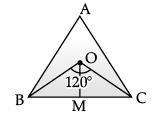i.e. ⇒ OM  = 16 cm. (∴ OB = 32 m)

Also, BM / OB = sin 60° = √3 / 2
i.e., BM = 16√3 cm
BC = 2BM = 32√3 cm.
Hence, area of ΔBOC = 1 / 2 BC.OM.
= 1 / 2 × 32√3 × 16
Area of ΔABC = 3 × area of ΔBOC
= 3 x 1 / 2 × 32√3 × 16
= 768√3 cm2.

(v) Find the area of shaded region (designed).  (1 Mark)
(a) 1900 cm2
(b) 1880.80 cm2
(c) 1888.104 cm2
(d) 1898.12 cm2
Ans. (c)
Solution. Area of shaded region (designed)
= Area of circle – Area of ΔABC
= (3218.28 – 768√3) cm2
= 1888.104 cm2.

Q.20. A pen stand made of wood is in the shape of a cuboid with four conical depressions to hold pens. The dimensions of the cuboid are 15 cm by 10 cm by 3.5 cm the radius of each of the depressions is 0.5 cm and the depth is 1.4 cm.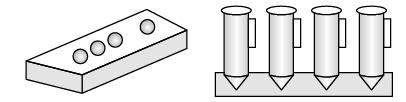(i) Find the diameter of each conical depression.   (1 Mark)
(a) 1 cm
(b) 1.5 cm.
(c) 2.0 cm
(d) 2.5 cm
Ans. (a)
Solution. Diameter of each conical depression = 2r
= 2 × 0.5 cm
= 1 cm.

(ii) Find the volume of the cuboid.  (1 Mark)
(a) 520 cm3
(b) 550 cm3
(c) 525 cm3
(d) 528 cm3
Ans. (c)
Solution. Volume of cuboid = l × b × h
= 15 cm × 10 cm × 3.5 cm
= 525 cm3.

(iii) Write the formula to find out the volume of conical depression.  (1 Mark)
(a) 1 / 2 πr2h
(b) 2πr2h
(c) πr2h
(d) 1 / 3 πr2h
Ans. (d)
Solution. Volume of conical depression = 1 / 3 πr2h

(iv) Find the volume of wood taken out to make four cavities.  (1 Mark)
(a) 30.5 cm3
(b) 30.2 cm3
(c) 32.5 cm3
(d) 1.47 cm3
Ans. (d)
Solution. Volume of wood taken out to make 4 cavities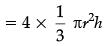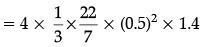= 1.47 cm3.

(v) Find the volume of the wood in the entire stand.  (1 Mark)
(a) 523.53 cm3
(b) 493 cm3
(c) 490 cm3
(d) 493.2 cm3
Ans. (a)
Solution. Volume of wood in the entire Stand
= Volume of cuboid – volume of 4 cavities
= 525 cm3 – 1.47 cm3
= 523.53 cm3.

Part – B

All questions are compulsory. In case of internal choices, attempt anyone.

Q.21. Find the zeroes of the quadratic polynomial x2 + 6x + 8.  (2 Mark)
OR
If zeroes of the polynomial x2 + 4x + 2a are a and 2 / α, then find the value of a.  (2 Mark)

Ans. p(x) = x2 + 6x + 8
As, p(x) = 0
x2 + 6x + 8 = 0
x2 + 4x + 2x + 8 = 0
x(x + 4) + 2(x + 4) = 0
(x + 4) (x + 2) = 0
∴ x = – 4 or – 2
∴ Roots are – 4 or – 2.
OR
Product of (zeroes) roots = c / a
= 2a / 1 = α. 2 / α
or, 2a = 2
∴ a = 1

Q.22. Find the value of k, for which the quadratic equation 2x2 + kx + 3 = 0 has equal roots.  (2 Mark)
OR
Find whether the lines represented by 2x + y = 3 and 4x + 2y = 6 are parallel, coincident or intersecting.  (2 Mark)

Ans. An equation has equal roots if the discriminant b2 − 4ac = 0
In the given equation,
2x2 + kx + 3 = 0
a = 2, b = k, c = 3
∴ Discriminant = k2 – 4(2)(3) = 0
= k2 – 24 = 0
∴ k2 = 24
or k = ± √24 = ± 2√6
OR
Here, a1 = 2, b1 = 1, c1 = – 3
and a2 = 4, b2 = 2, c2 = – 6
If a1 / a2 = b1 / b2 = c1 / c2,
then the lines are coincident.
Clearly, 2 / 4 = 1 / 2 = -3 / -6 = 1 / 2
Hence, lines are coincident.

Q.23. Find the number of natural numbers between 50 and 500 which are divisible by 7.  (2 Mark)
Ans. We observe that 56 is the first integer between 50 and 500 which is divisible by 7. Also, when we divide 500 by 7, the remainder is 3. Therefore, 500 − 3 = 497 is the largest integer divisible by 7 and lying between 50 and 500. Thus, we have to find the number of terms in an A.P. with first term = 56, last term = 497 and common difference = 7 (as the numbers are divisible by 7).
Let there be n terms in the A.P. Then, an = 497
an = a + (n −1) d
∴ a + (n − 1)d = 497
∴ 56 + (n − 1) × 7 = 497
[∵ a = 56 and d = 7]
∴ 7n + 49 = 497
∴ 7n = 448 or n = 64

Q.24. Find the ratio in which the y-axis divides the line segment joining the points (– 6, 7) and (3, 4). Also, find the coordinates of the point of division.  (2 Mark)
Ans. Let the point on the y-axis which divides the line segment joining the points A(– 6, 7) and B(3, 4) be C(0, y).
Let the ratio in which y-axis divides AB line segment be k : 1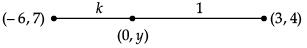By section formula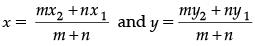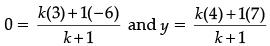∴ 3k – 6 = 0 or k = 2
∴ k : 1 = 2 : 1
and 4k +7 = y (k + 1)
(substitute value of k)
4(2) + 7 = y(2 + 1) 15
= 3y or y = 5
∴ (0, y) = (0, 5)
Thus, the line segment joining the points A(– 6, 7) and B(3, 4) is divided by the y-axis in the ratio 2 : 1 internally and the coordinates of the point of division is (0, 5).

Q.25. Find cosec 30° and cos 60° geometrically.  (2 Mark)
Ans.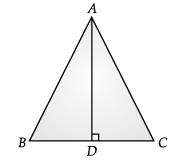Let a triangle ABC with each side equal to 2a.
∠A = ∠B = ∠C = 60°
ΔBDA ≌ ΔCDA by RHS
BD = CD
In ΔBDA, cosec 30° = AB / BD = 2a / a = 2
and cos 60° = BD / AB = a / 2a = 1 / 2

Q.26. Find the mode of the data using an empirical formula when it is given that mean is 30 and median is 25.  (2 Mark)
Ans. Given :
Mean = 30
Median = 25
Empirical formula is given by :
3 Median = Mode + 2 Mean
or, 3 × 25 = Mode + 2 × 30
or, 75 = Mode + 60
or, 75 – 60 = Mode
or, 15 = Mode
or, Mode = 15.

All questions are compulsory. In case of internal choices, attempt anyone.

Q.27. Three sets of English, Hindi and Sociology books dealing with cleanliness have to be stacked in such a way that all the books are stored topic wise and the height of each stack is the same. The number of English books is 96, the number of Hindi books is 240 and the number of sociology books is 336.   (3 Mark)
(i) Assuming that the books are of the same thickness, determine the number of stacks of English, Hindi and Sociology books.
(ii) Which mathematical concept is used in the problem ?

OR
Determine the values of m and n so that the following system of linear equations have infinite number of solutions :  (3 Mark)
(2m – 1)x + 3y – 5 = 0
and 3x + (n – 1)y – 2 = 0

Ans. (i) In order to arrange the books as required, we have to find the largest number that divides 96, 240 and 336 exactly, clearly, such a number is their HCF.
We have, By prime factorisation
96 = 25 × 3
240 = 24 × 3 × 5
and 336 = 24 × 3× 7
∴ HCF of  96, 240 and 336 is 24 × 3 = 48
So, there must be 48 books in each stack.
Number of stacks of English books
= 96 / 48 = 2
Number of stacks of Hindi books
240 / 48 = 5
Number of stacks of Sociology books
336 / 48 = 7
(ii) HCF of numbers.
OR
We have, for equation
(2m – 1)x + 3y – 5 = 0 ...(i)
a1 = 2m – 1, b1 = 3 and c1 = – 5
and for equation
3x + (n – 1)y – 2 = 0 ...(ii)
a2 = 3, b2 = (n – 1) and c2 = –2
For a pair of linear equations to have infinite number of solutions
a1 / a2 = b1 / b2 = c1 / c2
Or 2m - 1 / 3 = 3 / n - 1 = 5 / 2
or 2(2m –1) = 15 and 5(n – 1) = 6
Hence, m = 17 / 2 and n = 11 / 5

Q.28. Determine the AP whose 3rd term is 16 and 7th term exceeds 5th term by 12.  (3 Mark)
OR
A father’s age is three times the sum of the ages of his two children. After 5 years his age will be two times the sum of their ages. Find the present age of the father.  (3 Mark)

Ans. 7th term exceeds 5th term by 12
∴ a7 = a5 + 12
∴ a + 6d = a + 4d + 12
6d – 4d = 12
2d = 12 or d = 6,
As 3rd term = 16
∴ a3 = a + (n – 1)d
∴ a + 2 (6) = 16
a + 12 = 16
a = 4
∴ AP = 4 , 10 , 16.........
OR
Let the age of father be x years and sum of the ages of his children be y years.
After 5 years,
Father’s age = (x + 5) years
Sum of ages of his children = (y + 10) years
According to the given condition,
x = 3y ...(i)
and x + 5 = 2(y + 10)
or, x – 2y = 15 ...(ii)
Solving eqns. (i) and (ii), we get
3y – 2y = 15
⇒ y = 15
Substituting value of y in eq. (i), we get
x = 3 × 15 = 45
Hence, father ’s present age is 45 years.

Q.29. Find the point on x-axis which is equidistant from the points (2, – 5 ) and (– 2, 9).  (3 Mark)
Ans. Let the point on x axis is P(x, 0) which is equidistant from the points (2, – 5) and (– 2, 9).
The distance between PA is equal to PB such that, PA = PB
By distance formula,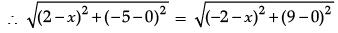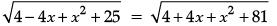– 4x + 25 = 4x + 81
8x = – 56 or x = – 7
So, the point P(–7, 0) is equidistant from the points (2, – 5) and (– 2, 9)

Q.30. Two tangents TP and TQ are drawn to a circle with centre O from an external point T. Prove that ∠PTQ = 2∠OPQ.  (3 Mark)
Ans.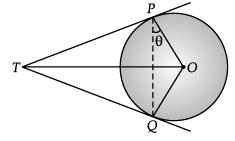Let ∠OPQ be θ.
∴ ∠TPQ = (90° – θ)
Since TP = TQ (Tangents)
∴ ∠TQP = (90° – θ)
(Opposite angles of equal sides)
Now, ∠TPQ + ∠TQP + ∠PTQ = 180°
⇒ 90° –  θ + 90° – θ + ∠PTQ = 180°
⇒ ∠PTQ = 180° – 180° + 2θ
⇒ ∠PTQ = 2θ
⇒ ∠PTQ = 2∠OPQ.

Q.31. If in a triangle ABC right angled at B, AB = 6 units and BC = 8 units, then find the value of sin A.cos C + cos A.sin C.  (3 Mark)
Ans. Here, AC= (8)2 + (6)2 = 100
or, AC = 10 units
(By Pythagoras Theorem)
∴ sin A = 8 / 10, cos A = 6 / 10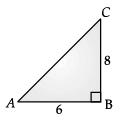and sin C = 6 / 10, cos C = 8 / 10
∴ sin A cos C + cos A sin C
= 8 / 10 x 8 / 10 + 6 / 10 x 6 / 10
= 64 / 100 + 36 / 100
= 100 / 100 = 1

Q.32. Find the area of the minor segment of a circle of radius 42 cm, if length of the corresponding arc is 44 cm.  (3 Mark)
Ans. Here, r = 42 cm
2πrθ / 360° = 44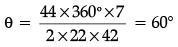Area of minor segment = area of sector – area of corresponding triangle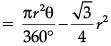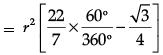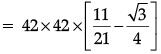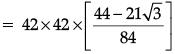= 21(44 – 21√3) cm2
= 21(44 – 36.37) cm2
= 21 × 7.63 cm2
= 160.23 cm2

Q.33. The table below show the salaries of 280 persons :   (3 Mark)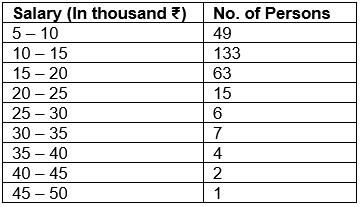Calculate the median salary of the data.
Ans.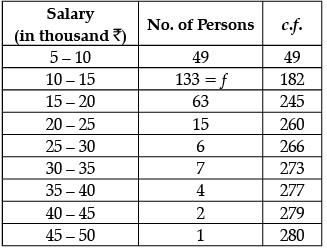N / 2 = 280 / 2 = 140.
Median class = 10 – 15.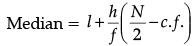= 10 + 5 / 133 (140 - 49)
= 10 +  5 x 91 / 133
= 13.42
Hence, Median salary is ₹ 13.42 thousand or ₹ 13420 (approx).

All questions are compulsory. In case of internal choices, attempt anyone.
Q.34. Find the value of a, b and c such that the numbers a, 9, b, 25, c are in A.P  (5 Mark)
Ans. Since a, 9, b, 25, c are in A.P.
Let common difference be d. a + d = 9 ...(i)
a + 3d = 25 ...(ii)
Solving (i) & (ii), we get
2d = 16 or d = 8
and a = 9 – 8 or a = 1

∴ Third term, b = a + 2d
= 1 + 2(8)
= 17
Also, fifth term, c = a + 4d
= 1 + 4(8)
= 33
So the AP is : 1, 9 ,17, 25 ,33

Q.35. Find the coordinates of the point which divide the line segment joining A(0, 5) and B(9, 2) into three equal parts.  (5 Mark)
OR
Which term of the Arithmetic Progression –7, –12, –17, –22, ... will be – 82 ? Is –100 any term of the A.P. ?
Ans. Let P(x1, y1) and Q(x2, y2) are two points which divide AB in three equal parts.
P divides AB in the ratio of 1 : 2 and Q divides AB in the ratio 2 : 1.
By section formula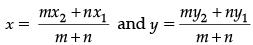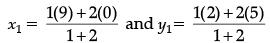x1 = 3 and y1 = 4
∴ P(x1, y1) = (3, 4)
Similarly, by section formula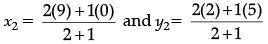∴ x2 = 6 and y2 = 3
∴ Q(x2, y2) = (6, 3)
OR
Given A.P. is
– 7, – 12, – 17, – 22, ......
Here, first term, a1 = – 7
second term, a2 = – 12
third term, a3 = – 17.
Common difference, d = a2 – a1 = a3 – a2 = – 12 – (– 7)= – 17 – ( – 12)
= – 12 + 7 = – 17 + 12
= – 5 = – 5
∴ d = – 5
Let an be the nth term of A.P. and it will – 82
i.e., an = – 82
Since, an = a1 + (n – 1) d
∴ – 82 = – 7 + (n – 1)(– 5)
⇒ – 82 = – 7 – 5(n – 1)
⇒ 82 = 5n + 2
⇒ 5n = 80
⇒ n = 16
Hence, 16th term of A.P. is – 82.
Since, these numbers are not factor of 5, hence – 100 will not be a term in the given A.P.

Q.36. Draw a circle of radius 3 cm. From a point P, 7 cm away from its centre draw two tangents to the circle. Measure the length of each tangent.  (5 Mark)
Ans.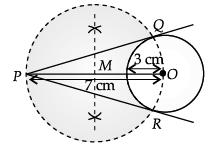Steps of construction :
1. Draw a line segment PO = 7 cm.
2. From the point O, draw a circle of radius = 3 cm.
3. Draw a perpendicular bisector of PO. Let M be the mid-point of PO.
4. Taking M as centre and OM as radius draw a circle.
5. Let this circle intersects the given circle at the point Q and R.
6. Join PQ and PR.
On measuring we get
PQ = PR = 6.3 cm

Offer running on EduRev: Apply code STAYHOME200 to get INR 200 off on our premium plan EduRev Infinity!

116 docs

,

,

,

,

,

,

,

,

,

,

,

,

,

,

,

,

,

,

,

,

,

;